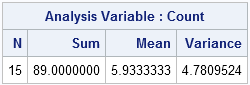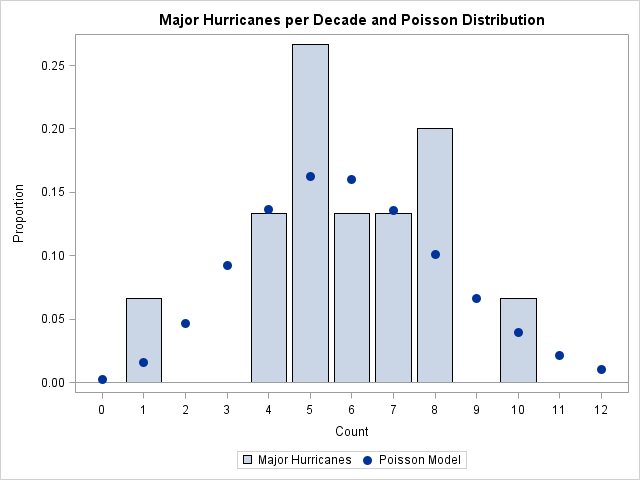Perhaps you saw the headlines earlier this week about the fact that it has been nine years since the last major hurricane (category 3, 4, or 5) hit the US coast. According to a post on the GeoSpace blog, which is published by the American Geophysical Union (AGU), researchers ran a computer model that "simulated the years 1950 through 2012 a thousand times to learn how often... virtual hurricanes managed to cross into 19 U.S. states ranging from Texas to Maine." Based on the results of their simulation study, the researchers concluded that it is highly unusual to have a nine-year period without major hurricanes: "Their analysis shows that the mean wait time before a nine-year hurricane drought occurred is 177 years." From that statement, I infer that the probability is 1/177 = 0.56% of having a nine-year "drought" in which major hurricanes do not hit the US.

Undoubtedly the simulation was very complex, since it incorporated sea temperatures, El Niño phenomena, and other climatic conditions. Is there an easy way to check whether their computation is reasonable? Yes! You can use historical data about major hurricanes to build a simple statistical model.

The National Hurricane Center keeps track of how many hurricanes of each Saffir-Simpson category hit the US mainland. The following SAS DATA step presents the data for each decade from 1851–2000:

```data MajorUS; input decade \$10. Count; datalines; 1851-1860 6 1861-1870 1 1871-1880 7 1881-1890 5 1891-1900 8 1901-1910 4 1911-1920 7 1921-1930 5 1931-1940 8 1941-1950 10 1951-1960 8 1961-1970 6 1971-1980 4 1981-1990 5 1991-2000 5 ; proc means data=MajorUS N sum mean var; var Count; run;```The call to PROC MEANS shows that 89 storms hit the coastal US in those 15 decades. That produces an average rate of λ = 5.93 major storms per decade. The very simplest statistical model of events that occur over time is a homogeneous Poisson process, which assumes that a major storm hits the US at a constant rate of 5.93 times per decade. Obviously that model is at best an approximation, since in reality El Niño and other factors affect the rate.

Nevertheless, this simple statistical model produces a result that is consistent with the more sophisticated computer model. In the Poisson model, we expect 0.9*5.93 = 5.337 major storms to hit land every nine years. Then the probability of observing zero storms in a nine-year period is the Poisson density evaluated at 0:

```/* During a 9 year period, we expect 0.9*5.9333333 major hurricanes. */ /* What is probability of 0 major hurricanes in a nine-year period? */ data _null_; lambda = 0.9*5.9333333; p = pdf("Poisson", 0, lambda); /* P(X=0) */ put p; run;```According to the model, a nine-year "drought" of major hurricanes occurs less than 0.5% of the time. That means that it will occur about once every 200 years. Recall that the model used by the climate scientists estimated 0.56% and 177 years.

How rare is this "drought" of major hurricanes at landfall? It is more unusual than tossing a fair coin and having it land on heads seven times in a row: 0.57 = 0.78%.

Considering that you can do the Poisson calculations on a hand calculator in about two minutes, the estimates are amazingly close to the estimates from the more sophisticated simulation model. Although a simple statistical model is not a replacement for meteorological models, it is a simple way to show the rarity of the current nine-year drought.

Postscript: Someone asked me how well these data fit a Poisson distribution. You can use the GENMOD procedure to fit a Poisson distribution in SAS. The procedure computes goodness-of-fit statistics that show that a Poisson model is a reasonable fit. For example, the deviance statistic is 12.97 which, for 14 degrees of freedom, give a p-value of 0.53 for the chi-square test of deviations from the Poisson model. Therefore the chi-square test does not reject the possibility that these data are Poisson distributed.

Personally, I often prefer graphs to p-values. The following graph overlays the Poisson model on the data. The fit is okay, but not stellar.Share